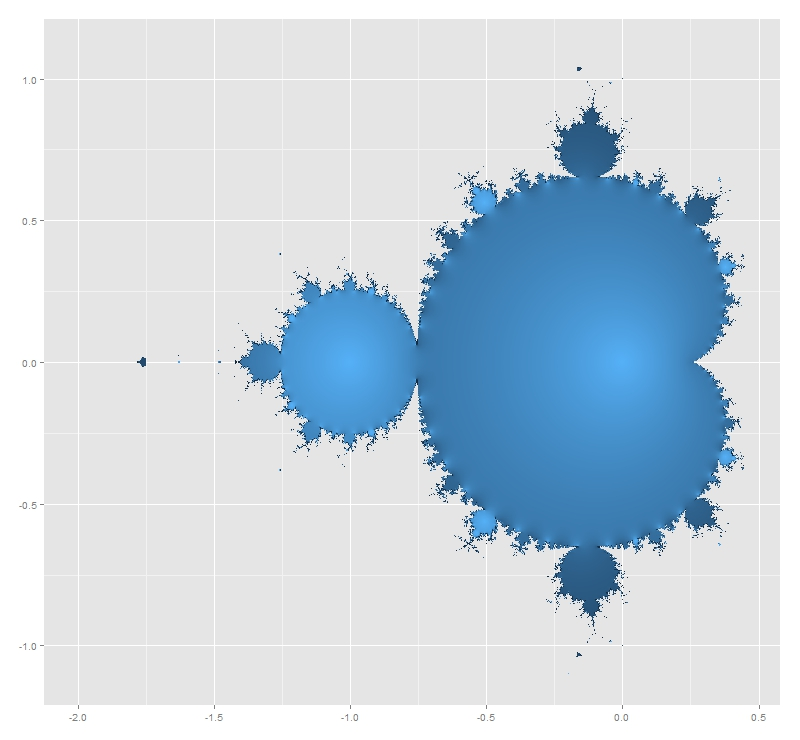# The Sound Of Mandelbrot Set

Music is the pleasure the human soul experiences from counting without being aware that it is counting (Gottfried Leibniz)

I like the concept of sonification: translating data into sounds. There is a huge amount of contents in the Internet about this technique and there are several packages in R to help you to sonificate your data. Maybe one of the most accessible is `tuneR`, the one I choosed for this experiment. Do not forget to have a look to `playitbyr`: a package that allows you to listen to a data.frame in R by mapping columns onto sonic parameters, creating an auditory graph, as you can find in its website. It has a very similar syntaxis to ggplot. I will try to post something about `playitbyr` in the future.

Let me start plotting the Mandelbrot Set. I know you have seen it lot of times but it is very easy to plot in with R and result is extremely beautiful. Here you have four images corresponding to 12, 13, 14 and 15 iterations of the set’s generator. I like a lot how the dark blue halo around the Set evaporates as number of iterations increases.

And here you have the Set generated by 50 iterations. This is the main ingredient of the experiment:Mandelbrot Set is generated by the recursive formula `xt+1=xt2+c`, with `x0=0`. A complex number c belongs to the Mandelbrot Set if its module after infinite iterations is finite. It is not possible to iterate a infinite number of times so every representation of Mandelbrot Set is just an approximation for a usually big amount of iterations. First image of Mandelbrot Set was generated in 1978 by Robert W. Brooks and Peter Matelski. You can find it here. I do not know how long it took to obtain it but you will spend only a couple of minutes to generate the ones you have seen before. It is amazing how computers have changed in this time!

This iterative equation is diabolical. To see just how pathological is, I transformed the succession of modules of `xt` generated by a given c in a succession of sounds. Since it is known that if one of this iterated complex numbers exceeds 2 in module then it is not in the Mandelbrot Set, frequencies of these sounds are bounded between 280 Hz (when module is equal to zero) and 1046 Hz (when module is equal or greater to 2). I called this function `CreateSound`. Besides the initial complex, you can choose how many notes and how long you want for your composition.

I tried with lot of numbers and results are funny. I want to stand out three examples from the rest:

• -1+0i gives the sequence 0, −1, 0, −1, 0 … which is bounded. Translated into music it sounds like an ambulance siren.
• -0.1528+1.0397i that is one of the generalized Feigenbaum points, around the Mandelbrot Set is conjetured to be self-similar. It sounds as a kind of Greek tonoi.
• -3/4+0.01i which presents a crazy slow divergence. I wrote a post some weeks ago about this special numbers around the neck of Mandelbrot Set and its relationship with PI.

All examples are ten seconds length. Take care with the size of the WAV file when you increase duration. You can create your own music files with the code below. If you want to download my example files, you can do it here. If you discover something interesting, please let me know.

Enjoy the music of Mandelbrot:

```# Load Libraries
library(ggplot2)
library(reshape)
library(tuneR)
rm(list=ls())
# Create a grid of complex numbers
c.points <- outer(seq(-2.5, 1, by = 0.002),1i*seq(-1.5, 1.5, by = 0.002),'+')
z <- 0
for (k in 1:50) z <- z^2+c.points # Iterations of fractal's formula
c.points <- data.frame(melt(c.points))
colnames(c.points) <- c("r.id", "c.id", "point")
z.points <- data.frame(melt(z))
colnames(z.points) <- c("r.id", "c.id", "z.point")
mandelbrot <- merge(c.points, z.points, by=c("r.id","c.id")) # Mandelbrot Set
# Plotting only finite-module numbers
ggplot(mandelbrot[is.finite(-abs(mandelbrot\$z.point)), ], aes(Re(point), Im(point), fill=exp(-abs(z.point))))+
geom_tile()+theme(legend.position="none", axis.title.x = element_blank(), axis.title.y = element_blank())
#####################################################################################
# Function to translate numbers (complex modules) into sounds between 2 frequencies
#   the higher the module is, the lower the frequencie is
#   modules greater than 2 all have same frequencie equal to low.freq
#   module equal to 0 have high.freq
#####################################################################################
Module2Sound <- function (x, low.freq, high.freq)
{
if(x>2 | is.nan(x)) {low.freq} else {x*(low.freq-high.freq)/2+high.freq}
}
#####################################################################################
# Function to create wave. Parameters:
#    complex     : complex number to test
#    number.notes: number of notes to create (notes = iterations)
#    tot.duration.secs: Duration of the wave in seconds
#####################################################################################
CreateSound <- function(complex, number.notes, tot.duration.secs)
{
dur <- tot.duration.secs/number.notes
sep1 <- paste(", bit = 16, duration= ",dur, ", xunit = 'time'),sine(")
sep2 <- paste(", bit = 16, duration =",dur,",  xunit = 'time'))")
v.sounds <- c()
z <- 0
for (k in 1:number.notes)
{
z <- z^2+complex
v.sounds <- c(v.sounds, abs(z))
}
v.freqs <- as.vector(apply(data.frame(v.sounds), 1, FUN=Module2Sound, low.freq=280, high.freq=1046))
eval(parse(text=paste("bind(sine(", paste(as.vector(v.freqs), collapse = sep1), sep2)))
}
sound1 <- CreateSound(-3/4+0.01i     , 400 , 10) # Slow Divergence
sound2 <- CreateSound(-0.1528+1.0397i, 30  , 10) # Feigenbaum Point
sound3 <- CreateSound(-1+0i          , 20  , 10) # Ambulance Siren
writeWave(sound1, 'SlowDivergence.wav')
writeWave(sound2, 'FeigenbaumPoint.wav')
writeWave(sound3, 'AmbulanceSiren.wav')
```